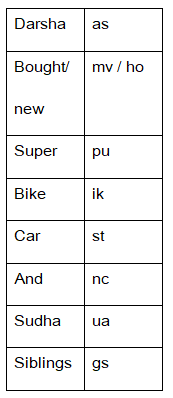# NIACL AO Prelims – Reasoning Ability Questions 2021 (Day-02)

Dear Aspirants, Our IBPS Guide team is providing new series of Reasoning Questions for NIACL AO Prelims 2021 so the aspirants can practice it on a daily basis. These questions are framed by our skilled experts after understanding your needs thoroughly. Aspirants can practice these new series questions daily to familiarize with the exact exam pattern and make your preparation effective.

Start Quiz

Number series:

Direction (1-4): Study the following information carefully and answer the below questions.

214 429 792 643 943

1) If all the digits of the numbers are written in descending order within the number, then which of the following number will be the second-highest number?

A.429

B.792

C.643

D.943

E.214

2) If the first digit of the lowest number is multiplied by the second digit of the second-lowest number then the results should be divided by the second digit of the highest number, which of the following number is obtained?

A.4

B.3

C.2

D.1

E.6

3) If we interchange the position of the first and last digit of the numbers, then how many numbers thus formed will be divisible by three?

A.1

B.2

C.3

D.4

E.None

4) If we add the first and last digit of the number and the resultant is subtracted from the second digit of the number, then which of the following number gives a resultant as the highest number?

A.943

B.643

C.792

D.214

E.429

Miscellaneous

5) If 1 is subtracted from all the even digits and 2 is added to all the odd digits of the number “587412463257”, then which of the following numbers are repeated more than twice?

A.3

B.9

C.5

D.Both a and e

E.7

Coding decoding

Direction (6-9): Study the following information carefully and answer the below questions.

In a certain code language

“Darsha bought new car” is written as “as ho mv st”,

“Darsha and Sudha Siblings” is written as “ua as nc gs”,

“Sudha bought new bike” is written as “ua mv ho ik”,

“Car and Bike Super” is written as “ik nc st pu”

6) What could be the code for “Bought”?

A.mv

B.ho

C.pu

D.st

E.Can’t be determined

7) Which of the following is the code for “ Darsha Bike Super”?

A.gs ik nc

B.as ik pu

C.ho ik as

D.pu ua nc

E.st pu nc

8) Which of the following can represent the code ”st gs ik“?

A.new bought super

B.Car new siblings

C.Car siblings Bike

D.Sudha siblings bike

E.None of these

9) If “New Dress” is coded as “mv dx”, then what is the code for “bought”?

A.gs

B.nc

C.mv

D.ho

E.pu

#### Inequality

10) In which of the following expressions will the expression L > P will be False?

A.L > M ≥ N = P

B.P = N ≥ L > M

C.P ≤ M ≤ N < L

D.L > M = N ≥ P

E.None of these

### Try NIACL AO Online Practice Mock Test

214 429 792 643 943

421 942 972 643 943

214 429 792 643 943

2*2=4/4=1

214 429 792 643 943

412 924 297 346 349

214 429 792 643 943

5  11  0  5  8

587412463257

779331355179

Directions (6-9) :In option 1, L > M ≥ N = P, L > P is true

In option 2, P = N ≥ L > M, P ≥ L so L > P is false

In option 3, P ≤ M ≤ N < L, L > P is true

In option 4, L > M = N ≥ P, L > P is true

Hence, option b.